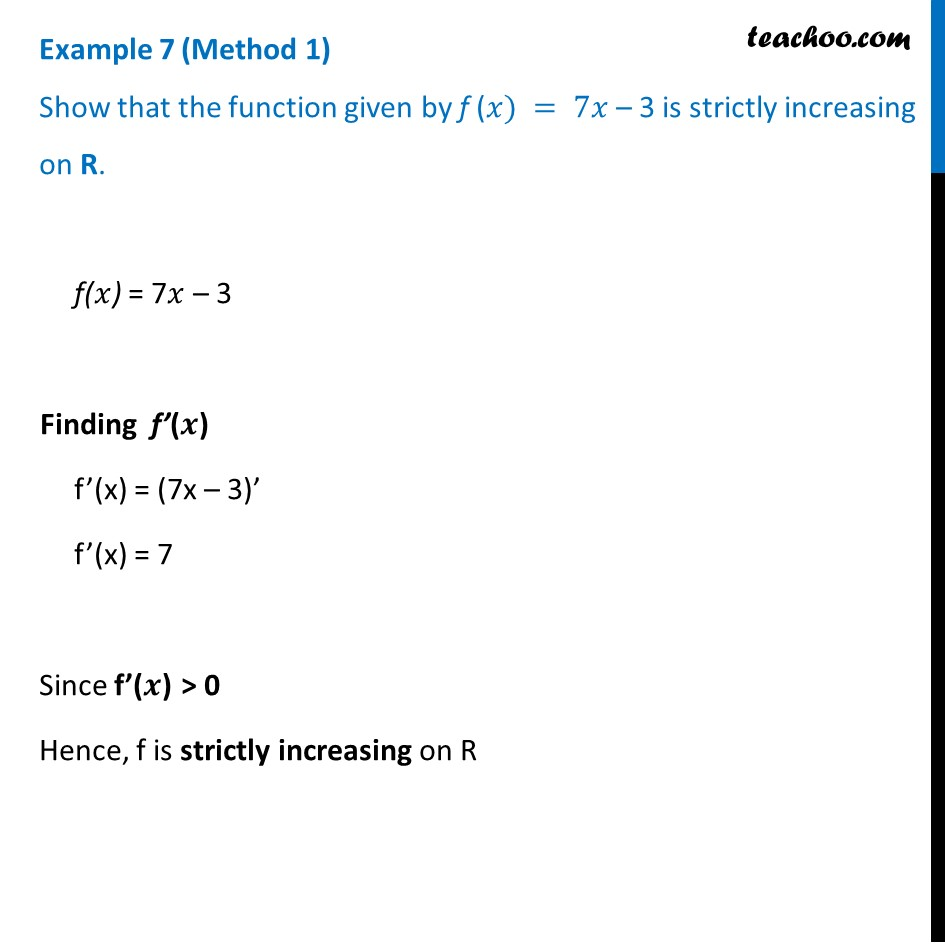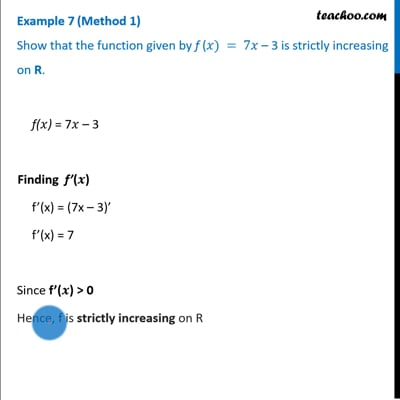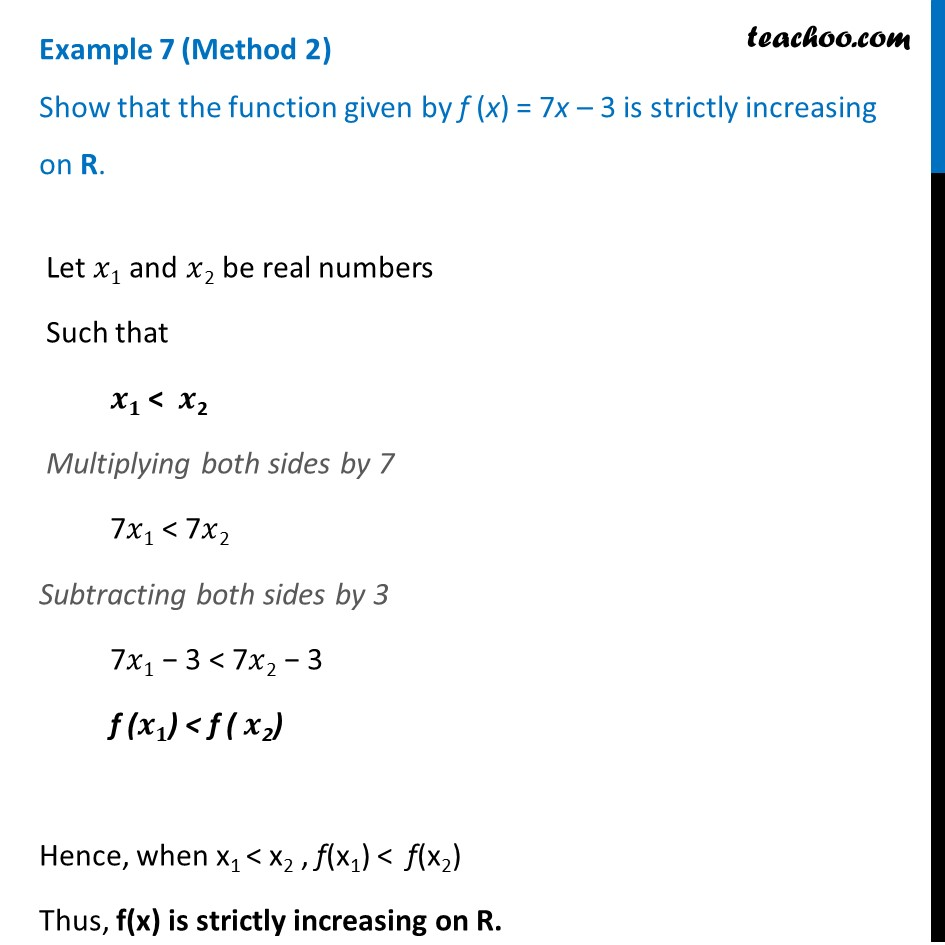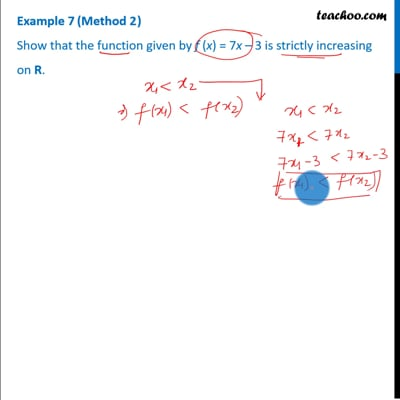Examples

Chapter 6 Class 12 Application of Derivatives
Serial order wiseThis video is only available for Teachoo black usersThis video is only available for Teachoo black users

Solve all your doubts with Teachoo Black (new monthly pack available now!)

### Transcript

Example 7 (Method 1) Show that the function given by f (𝑥) = 7𝑥 – 3 is strictly increasing on R.f(𝑥) = 7𝑥 – 3 Finding f’(𝒙) f’(x) = (7x – 3)’ f’(x) = 7 Since f’(𝒙) > 0 Hence, f is strictly increasing on R Example 7 (Method 2 ) Show that the function given by f (x) = 7x – 3 is strictly increasing on R. Let 𝑥1 and 𝑥2 be real numbers Such that 𝒙𝟏 < 𝒙2 Multiplying both sides by 7 7𝑥1 < 7𝑥2 Subtracting both sides by 3 7𝑥1 − 3 < 7𝑥2 − 3 f (𝒙𝟏) < f ( 𝒙2) Hence, when x1 < x2 , f(x1) < f(x2) Thus, f(x) is strictly increasing on R.